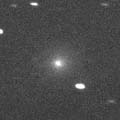# \$BK\ED(B-\$B%`%k%3%9(B-\$B%Q%\$%I%%%7%c!<%3%t%!!

45P/Honda-Mrkos-Pajdusakova (2006)###\$B###\$B50F;MWAG(B

```The following orbital elements are taken from MPC 56957:

Epoch 2006 July 4.0 TT = JDT 2453920.5
T 2006 June 29.8039 TT                                  MPC
q   0.530256             (2000.0)            P               Q
n   0.1876368      Peri.  326.1196      +0.5687544      -0.8191580
a   3.021741       Node    89.1100      +0.7701270      +0.4987067
e   0.824520       Incl.    4.2531      +0.2888302      +0.2833229
P   5.25
From 86 observations 2000-2006, mean residual 0".8.
```

###\$B@1?^(B###\$B8wEYJQ2=(B

```        m1 = 16.0 + 5 log\$B&\$(B + 30.0 log r  [ ,0]  (              \$B!A(B2006\$BG/(B 6\$B7n(B29\$BF|(B)
m1 = 12.0 + 5 log\$B&\$(B + 15.0 log r  [0, ]  (2006\$BG/(B 6\$B7n(B29\$BF|!A(B              )
```##### \$B50F;MWAG\$O(BMPC\$B\$N(BEphemerides and Orbital Elements\$B\$K7G:\\$5\$l\$?\$b\$N\$G\$9!#(B \$B@1?^\$O(B StellaNavigator Ver.2.0 for Windows (\$B%"%9%H%m%"!<%D(B \$BJTCx(B / \$B%"%9%-!<=PHG6I4)(B) \$B\$G:n@.\$7\$?\$b\$N\$G\$9!#(B \$B8wEY%0%i%U\$O(BComet for Windows\$B\$G:n@.\$7\$?\$b\$N\$G\$9!#(B